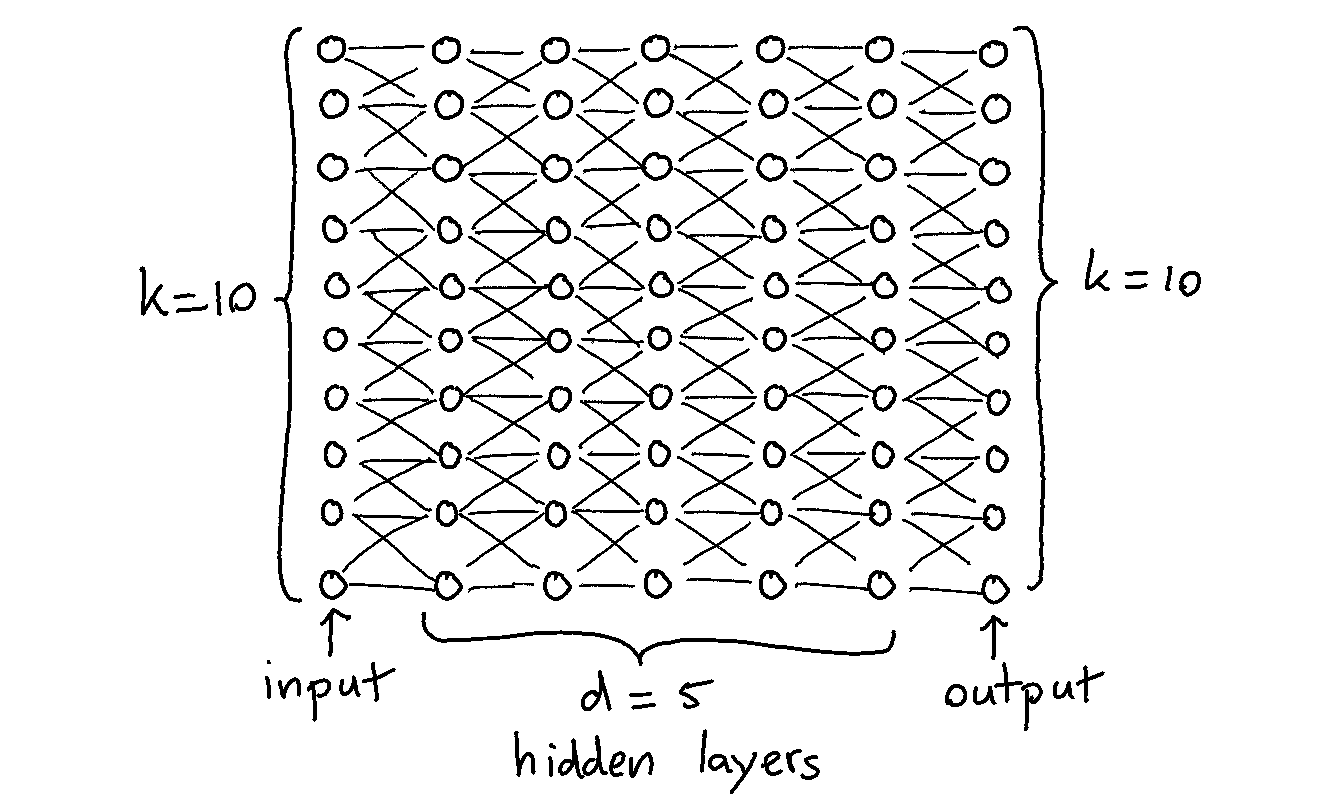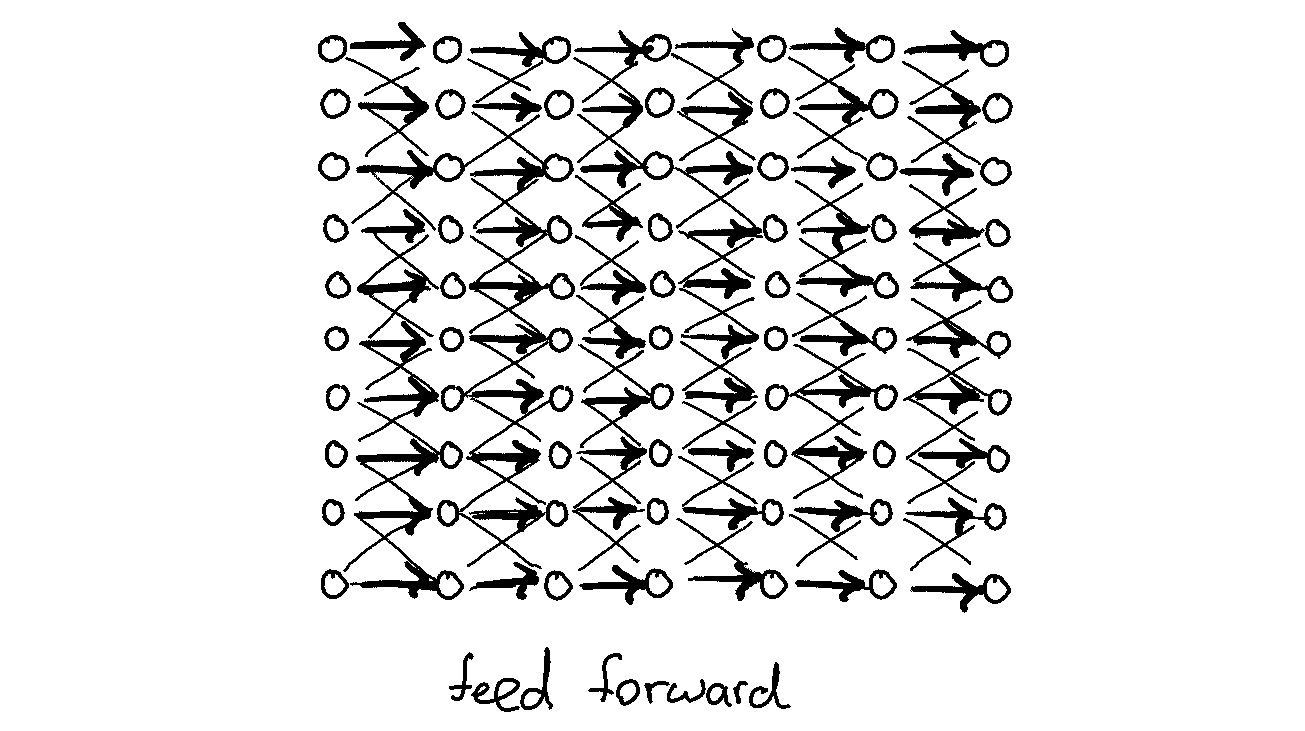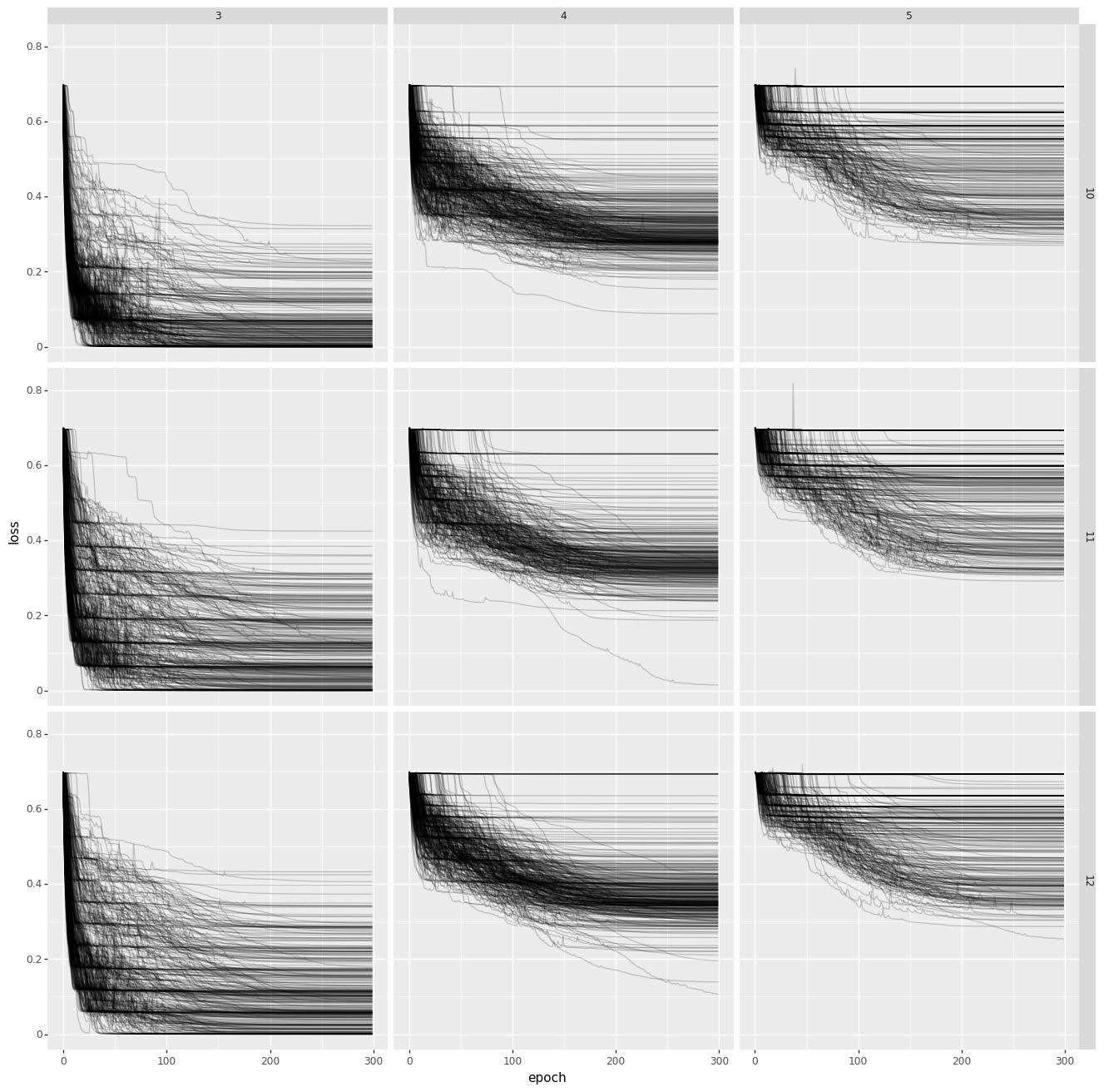# Parallel Grid

An Ode to Pipes, Seeds and Simplicity

Vincent Warmerdam koaning.io
11-16-2019

One reason to use scikit-learn is to have access to a GridSearchCV object that can run tasks in parallel. But what about things that don’t fit the api? There’s keras/pytorch that needs custom code before it works nicely but there’s also tasks in fields like operations research/heuristics that won’t fit too swell.

I was thinking about a nice way to abstract this and came to the conclusion that this is something you probably don’t want to solve with python. Instead you can solve this from the command line with parallel.

## Usecase

The reason I had this usecase was because I was wondering what the effect of a seed is when training a neural network. I don’t like the idea of having a seed as a hyperparameter but I don’t mind having a measure of risk. If the algorithm depends a lot on the seed then this would be a reason not to pick it.

The setup was simple: train a neural network that needs to learn to be the identity function. I decided to generate a dataset with $$k$$ columns containing zeros and ones. The neural network would have $$d$$ hidden layers that are fully connected and each layer has $$k$$ nodes. Below is a drawing for $$k=10$$ and $$d=5$$.The reason why these networks are interesting to train for is because they should theoretically be able to always achieve a loss function of zero. The network merely needs to learn the identity function so it only needs to propogate zeros and ones forward.Still, this is something that will have a random seed so I’ll need to run this many times. This got me wondering about what might be the most convenient approach.

## Parellism

From the terminal you can create a sequence appear.


> seq 5
1
2
3
4
5

This output can be piped into another command. In particular it can be piped to the parallel command. This command will run another command from the command line in parallel. Here’s the syntax.


seq <numjobs> | parallel -j <threads> <command>

For example. Suppose that I have a job in a python file called main.py which will sample random grid parameters, run an experiment, and log the output to a file. Then this command will run that 1000 times while using 4 threads.


seq 1000 | parallel -j 4 python main.py

### Random Python Jobs

The main.py job can be described using randomness.


res = build_train({'num_columns': random.choice([10, 11, 12]),
'num_layers': random.choice([1, 2, 3, 4, 5]),
'loss_func': 'binary_crossentropy',
'tf_seed': random.randint(1, 4200),
'epochs': 300,
'rows': random.choice([2000, 3000, 4000])})
with open(f"/tmp/gridlogs/{str(uuid.uuid4())[:14]}.jsonl", "w") as f:
json.dump(res, f)

When you run it with this:


> seq 500 | parallel -j 6 python main.py

Then you’ll run main.py 500 times while having running 6 jobs at a time. But you’re not just limited to random search.

## Using Itertools

You could also make it deterministic. Take this jobfile;


# capture.py
import sys

if __name__ == "__main__":
print(f"i am seeing this input: {sys.argv}")

From python we can capture the that comes out of seq.


> seq 5 | parallel -j 12 python capture.py
i am seeing this input: 1
i am seeing this input: 2
i am seeing this input: 3
i am seeing this input: 4
i am seeing this input: 5

This also means that we can user an iterator to generate a grid and we can have the input determine which settings to select. Example below;


import sys
import time
import itertools as it

def mkgrid():
for param_a in range(8):
for param_b in range(8):
yield {"param_a": param_a, "param_b": param_b}

grid = mkgrid()

if __name__ == "__main__":
settings = it.islice(grid, int(sys.argv), int(sys.argv) + 1)
time.sleep(1)
print(f"these are the settings: {next(settings)}")

You’ll need to tell seq to start at 0 and end at 63 but that’s about it. This job takes 8 seconds if you have enough cores:


> seq 0 63 | parallel -j 8 python capture.py

## Benefits

This approach works pretty well on my mac. It comes with a few cores built in and it’s usually not doing anything during the evening. This approach generated a dataset with a million rows overnight. It might be enough for your jobs too without the need for a cloud vendor.

Also note that you can just log to a random file on a set path on disk. No locking of a single file between processes. There’s also no notebook and just a single main.py file which can still be unit tested.

I also enjoy the feeling that I don’t need to worry about parallism from python. This is just something that the operating systems handles for me now from the command line. Win!

## Results

The code can be found in this gist and the main result is summerised in the next chart.

The chart shows the loss over the epochs during training. Each line is a seperate run. The grid shows the number of hidden layers (3, 4, 5) and the number of inputs (10, 11, 12). Notice that not few training procedures converge to zero loss even though this is certainly possible to achieve.When an algorithm depends so much on the seed, you start wondering if it’s a good idea to fully trust it. It should be said that this phenomenon mainly occurs for very deep neural networks not shallow ones and the example is a bit theoretical. Neural networks with just one hidden layers tend to converge immediately.